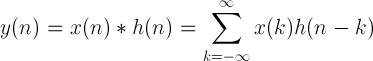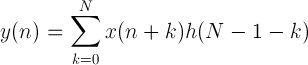# Convolution calculator

 Enter first data sequence: 1 1 1 0 0 0 Enter second data sequence: 0.5 0.2 0.3 Result data sequence:

## Convolution calculation

The sequence y(n) is equal to the convolution of sequences x(n) and h(n):For finite sequences x(n) with M values and h(n) with N values:For n = 0 .. M+N-2